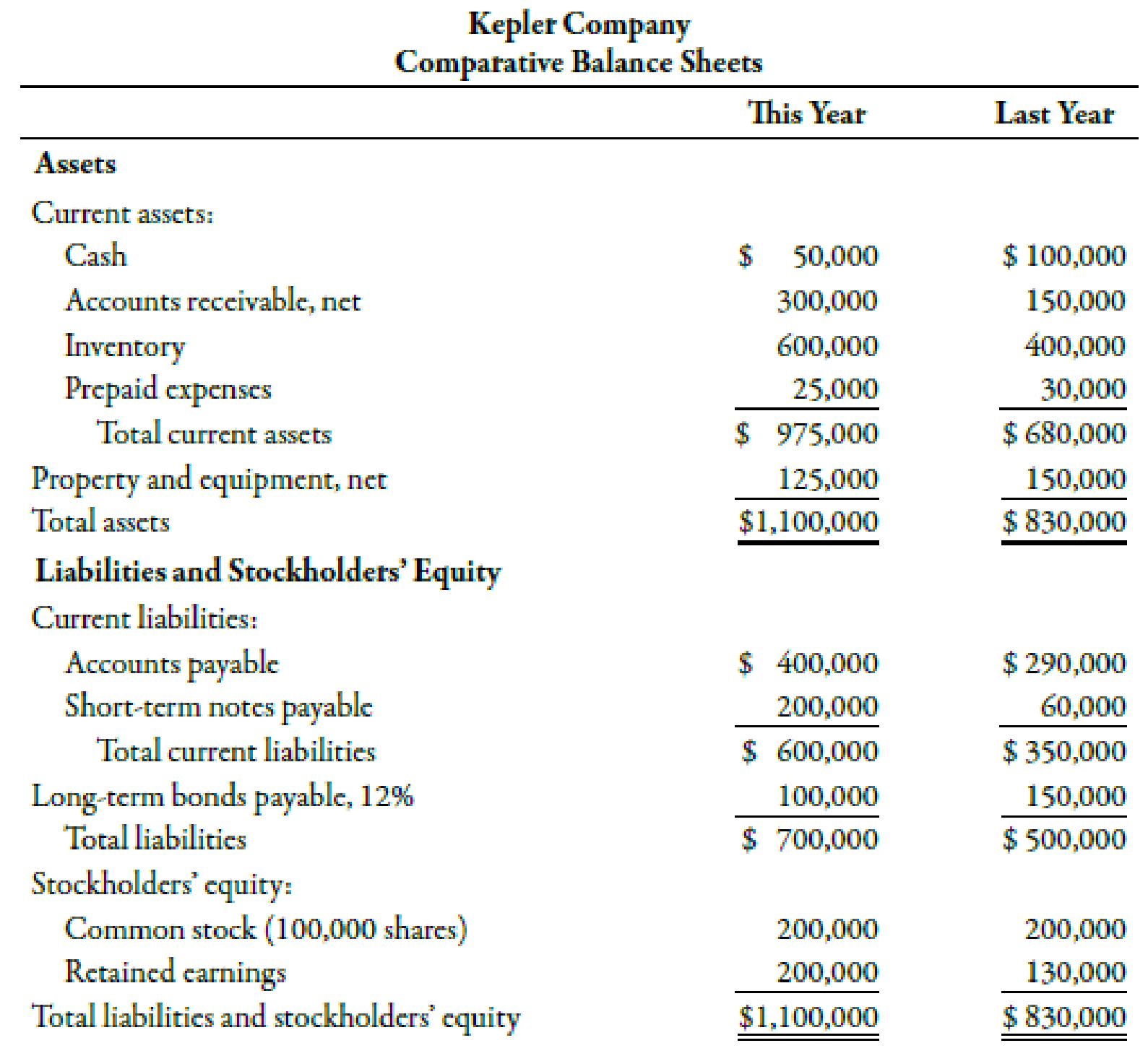# Mike Sanders is considering the purchase of Kepler Company, a firm specializing in the manufacture of office supplies. To be able to assess the financial capabilities of the company, Mike has been given the company’s financial statements for the 2 most recent years. Refer to the information for Kepler Company on the previous page. Required: Note : Round all answers to two decimal places. 1. Compute the following for each year: (a) the times-interest-earned ratio and (b) the debt ratio 2. CONCEPTUAL CONNECTION Does Kepler have too much debt? What other information would help in answering this question?### Managerial Accounting: The Corners...

7th Edition
Maryanne M. Mowen + 2 others
Publisher: Cengage Learning
ISBN: 9781337115773

#### Solutions

Chapter
Section### Managerial Accounting: The Corners...

7th Edition
Maryanne M. Mowen + 2 others
Publisher: Cengage Learning
ISBN: 9781337115773
Chapter 15, Problem 62P
Textbook Problem
25 views

## Mike Sanders is considering the purchase of Kepler Company, a firm specializing in the manufacture of office supplies. To be able to assess the financial capabilities of the company, Mike has been given the company’s financial statements for the 2 most recent years.Refer to the information for Kepler Company on the previous page.Required:Note: Round all answers to two decimal places. 1. Compute the following for each year: (a) the times-interest-earned ratio and (b) the debt ratio 2. CONCEPTUAL CONNECTION Does Kepler have too much debt? What other information would help in answering this question?

1.

To determine

Calculate times interest earned ratio and debt ratio.

### Explanation of Solution

Times-Interest-Earned Ratio:

Times-interest-earned ratio is a leverage ratio that determines the firm’s ability to pay its debt by assessing the income statement of the firm. It is calculated by the addition of income before tax and interest expense and dividing the summation amount by interest expense.

Debt Ratio:

Debt ratio is a leverage ratio that evaluates the proportion of total liabilities to total assets. The ratio measures the extent to which creditors are protected for insolvency.

(a)

Use the following formula to calculate the value of times-interest-earned ratio of this year:

Times-Interest-Earned Ratio=Income before Tax+Interest ExpenseInterest Expense

Substitute $163,000 for income before tax and$12,000 for interest expense in the above formula.

Times-Interest-Earned Ratio=$163,000+$12,000$12,000=14.58 times Therefore, the value of times-interest-earned ratio for this year is 14.58 times. Use the following formula to calculate the value of times-interest-earned ratio of last year: Times-Interest-Earned Ratio=Income before Tax+Interest ExpenseInterest Expense Substitute$132,000 for income before tax and $18,000 for interest expense in the above formula. Times-Interest-Earned Ratio=$132,000+$18,000$18,000=8

2.

To determine

Identify whether Company KE have too much debt. Also, identify the other needed information to explain if company KE has too much debt.

### Still sussing out bartleby?

Check out a sample textbook solution.

See a sample solution

#### The Solution to Your Study Problems

Bartleby provides explanations to thousands of textbook problems written by our experts, many with advanced degrees!

Get Started

Find more solutions based on key concepts
Use a production possibilities frontier to describe the idea of efficiency.

Principles of Microeconomics (MindTap Course List)

CHECKING ACCOUNT TERMS Match the following words with their definitions:

College Accounting, Chapters 1-27 (New in Accounting from Heintz and Parry)

If the United States imports more goods from abroad than it exports, foreigners will tend to have a surplus of ...

Fundamentals of Financial Management, Concise Edition (with Thomson ONE - Business School Edition, 1 term (6 months) Printed Access Card) (MindTap Course List)

What does the invisible hand of the marketplace do?

Principles of Macroeconomics (MindTap Course List)